# What is Acceleration?

• Last Updated : 09 Aug, 2021

When a stationary automobile accelerates rapidly, we are pushed backward; when the brakes are used, we are pushed forward against our seat; and when our automobile makes a quick right turn, we are pushed to the left. We are in these circumstances because our automobile is speeding up. As such, acceleration occurs whenever there is a change in velocity. Let’s look at some instances to help you comprehend the notion of acceleration.

Assume a person has a car that is traveling in a straight path at a constant speed of 10 km/h while a helicopter is noticed flying at around 26 km/h. If someone asked you, where do you locate the acceleration in these two cases? Your response will undoubtedly be no because both are going at the same speed, implying that no acceleration exists in both cases.

Hey! Looking for some great resources suitable for young ones? You've come to the right place. Check out our self-paced courses designed for students of grades I-XII

Start with topics like Python, HTML, ML, and learn to make some games and apps all with the help of our expertly designed content! So students worry no more, because GeeksforGeeks School is now here!

### What is the Motion in a Straight Line?

Motion in a straight line refers to the displacement of an object with respect to time while the object moves along a straight path. It is a uni-dimensional motion and can be well expressed using the X-axis coordinate system alone. This is also termed Linear Motion.

e.g. A car moving along the linear path in a uniform direction.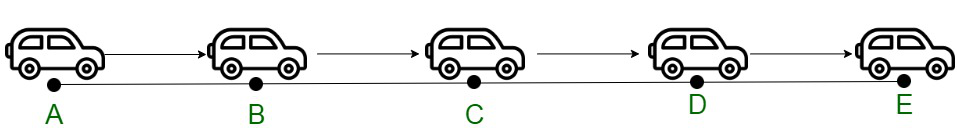Following are the types of motion in a straight line:

1. Uniform Motion – The uni-dimensional motion of an object where it travels with uniform speed all along the path is called Uniform motion. Since the body covers equal distances in equal intervals of time, the velocity of the body remains constant. The speed of an object remains the same during all time frames, the average speed of the object is equivalent to its actual speed. No acceleration is attained by the object in the case of uniform motion. For instance, a car traveling 20 km in the first hour, 20 km in the next hour, and so on continuing with this throughout its motion.

Examples:

• The hands of the clock cover equal distances.
• A car going along a straight level road at a steady speed.
• An airplane flying at a steady speed in the air.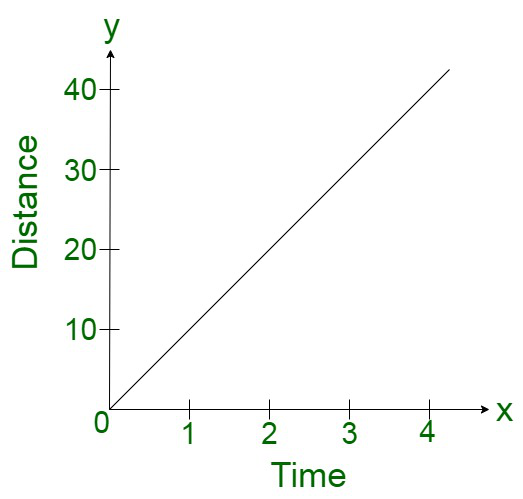Distance Time Graph for Uniform Motion

2. Non-uniform motion in a straight line (Accelerated motion) – The uni-dimensional motion of an object where it travels with a varying speed all along the path is called Non-Uniform motion. Since the body covers unequal distances in equal intervals of time, the velocity of the body remains modified. The speed of an object changes during the time frames, the average speed may be different from its actual speed. Acceleration or deceleration is attained by the object in the case of non-uniform motion. For instance, a car traveling 20 km in the first hour, 30 km in the next hour, and so on continuing with a varying speed throughout its motion.

Examples:

• A racing person.
• A ball bouncing at different intervals
• Two cars colliding with each other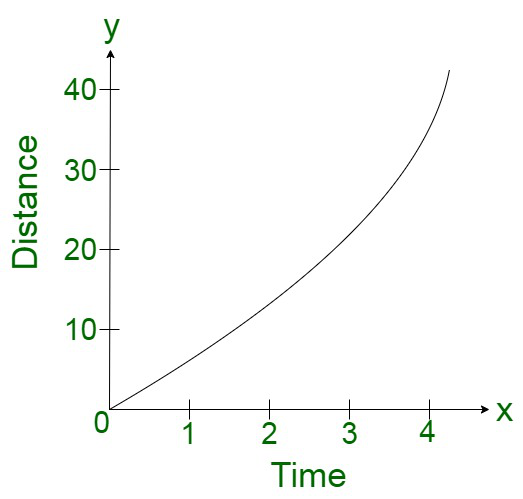Distance Time Graph for Non-Uniform Motion

### Acceleration

Acceleration is termed as the rate of change of velocity which is computed as a function of time. Or in other words, the acceleration can be visualized as the second derivative of position concerning time.

It is a vector quantity, which is associated with both magnitude and direction. It is denoted by ‘a’.

The unit of acceleration is meters per second squared or meters per second (the object’s speed or velocity) per second or m/s2.

The dimensional formula of acceleration is [M0 L1 T-2].

Acceleration can be positive, zero, or negative. In case the object’s velocity increases with time, it can be termed as Positive Acceleration. In case the velocity is zero, it is termed Zero Acceleration, while the negative acceleration is also known as retardation indicates a decrease in velocity with time.

Mathematically, the change in the velocity of an object in motion is defined as, (v – u) where v and u are the final and the initial velocities.

Therefore, the acceleration of the object is given by,

Acceleration = Change in velocity / Time taken

or

a = (v – u) / t

where t is the time taken by the object.

### Types of Acceleration

Following are the different types of acceleration associated with an object as,

1. Average Acceleration: The average acceleration is defined as the change in velocity for a particular specified time interval. The average acceleration can be calculated for a time instance, as follows,

av = Δ v / Δ t

or

av = (vf – vi) / (tf – ti)

where vf is the final velocity, vi is the initial velocity, ti is the initial time and tf is the final time.

2. Instantaneous Acceleration:  In order to calculate the instantaneous acceleration, the average velocity can be computed between two points in time separated by Δt and let Δt approach zero. The result obtained is the derivative of the velocity function v(t), which is instantaneous acceleration. Mathematically,Thus, similar to velocity being the derivative of the position function, instantaneous acceleration is the derivative of the velocity function. We can show this graphically in the same way as instantaneous velocity. In (Figure), instantaneous acceleration at time t0 is the slope of the tangent line to the velocity-versus-time graph at time t0. We see that average acceleration given as,Approaches instantaneous acceleration as Δt approaches zero. Also in part (a) of the figure, instantaneous acceleration is shown for the point on the velocity curve at maximum velocity. With a slope value of 0, the maximum velocity is attained. This time corresponds to the zero of the acceleration function. In part (b), instantaneous acceleration at the minimum velocity is zero, since the slope of the curve is zero there, too.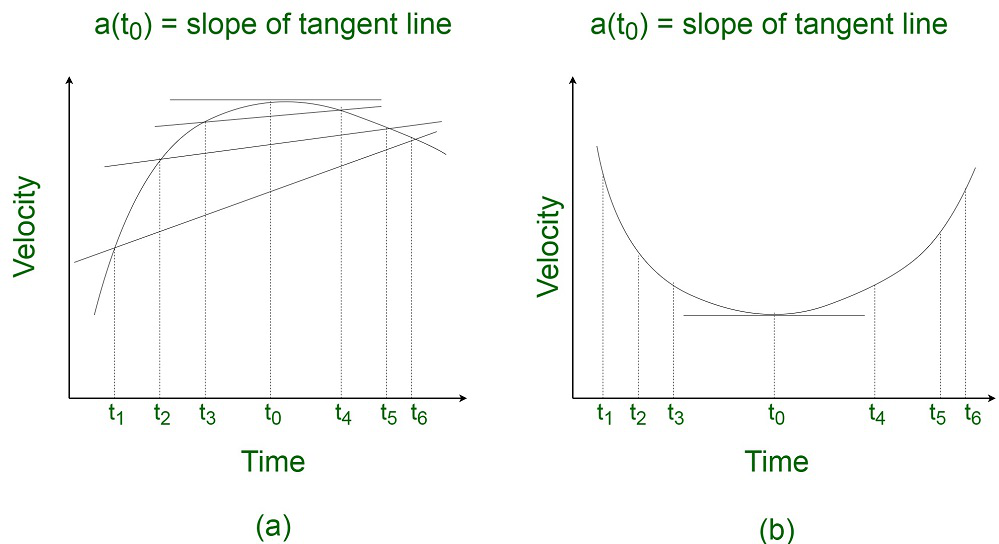In the velocity-time graph, instantaneous acceleration is equivalent to the slope of the tangent line.

(a) The average acceleration:Δt = t0 – t1, Δt = t5 – t2 and Δt = t4 – t3

When t ⇢ 0. We can conclude that the average acceleration approaches instantaneous acceleration at time t0

Thus, for a given velocity-time function, the zero of the acceleration function is attained either at a minimum or the maximum velocity.

Various graphs related to Motion (For Acceleration)

Displacement-Time graph

In this graph time is plotted on x-axis and displacement on y-axis. In case of an accelerated motion (a > 0) , the slope of this graph increases with time, where as for decelerated motion, the slope decreases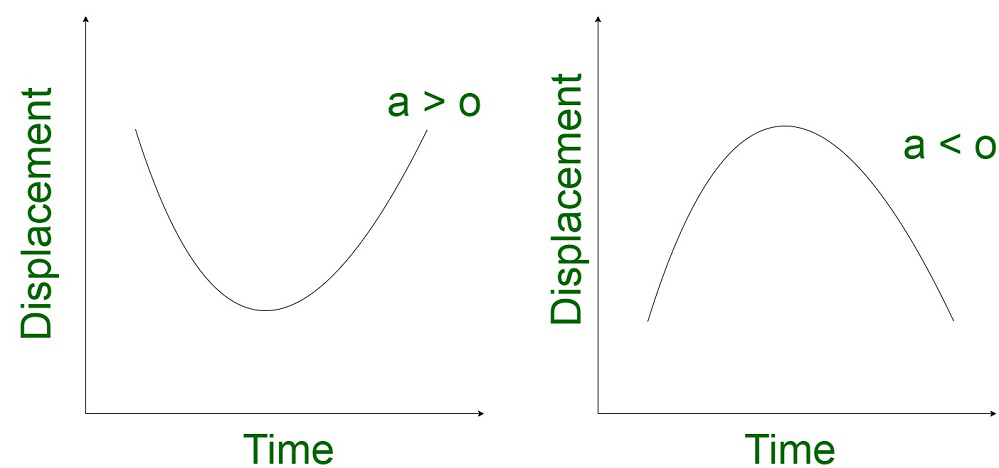Velocity-Time graph

The curves indicate the velocity-time graph time is plotted along x-axis and velocity is plotted along y-axis. While finding displacement through v – t graph, keeping sign under consideration.

• When the velocity of the particle is constant or acceleration is zero.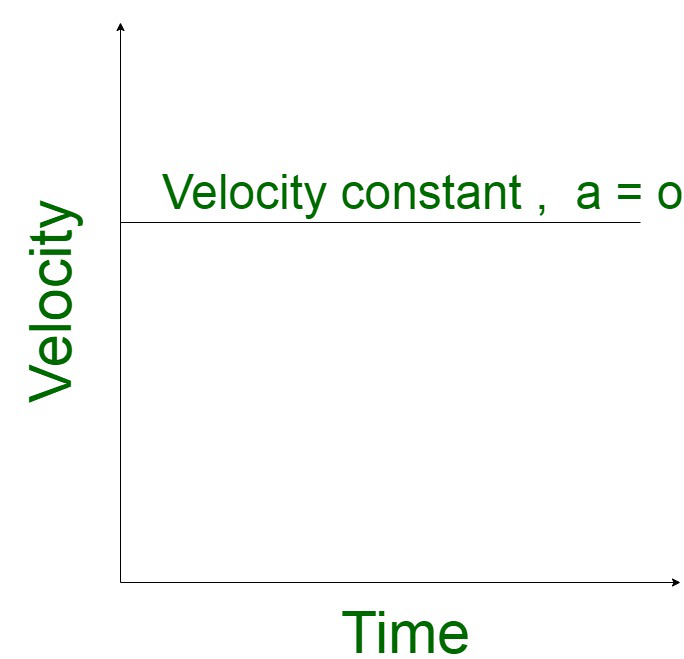• When the particle is moving with a constant acceleration and its initial velocity is zero.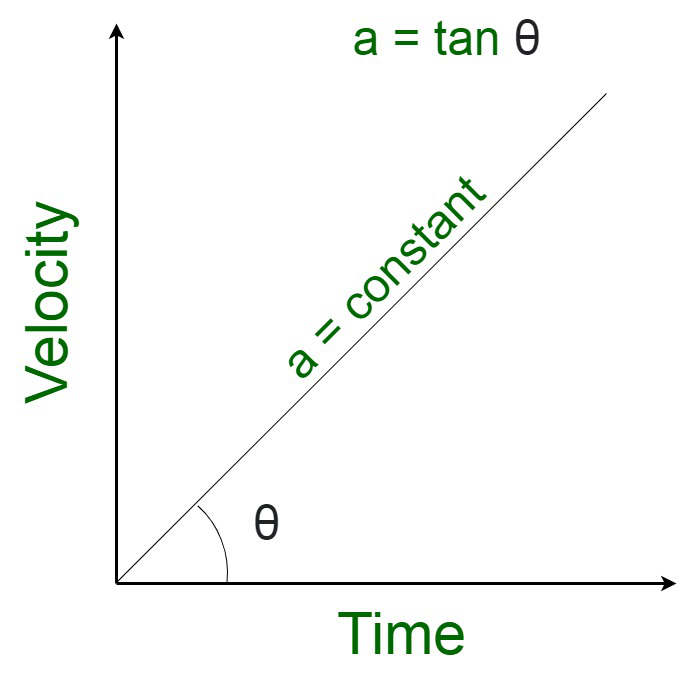• When the particle is moving with constant retardation.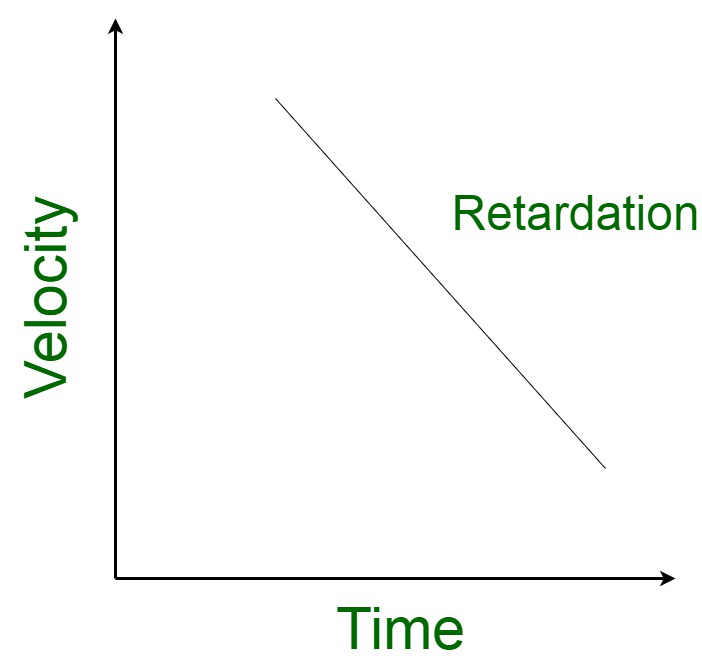• When the particle moves with non-uniform acceleration and its initial velocity is zero.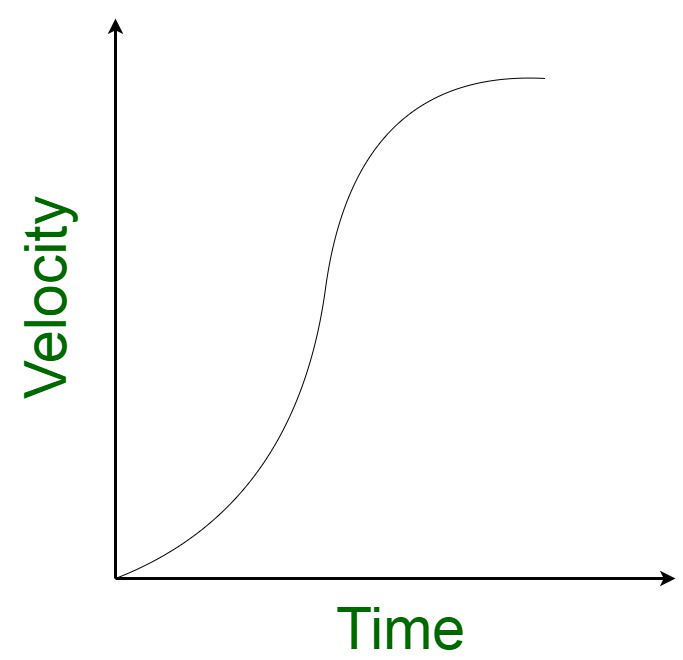• When the acceleration decreases and increases.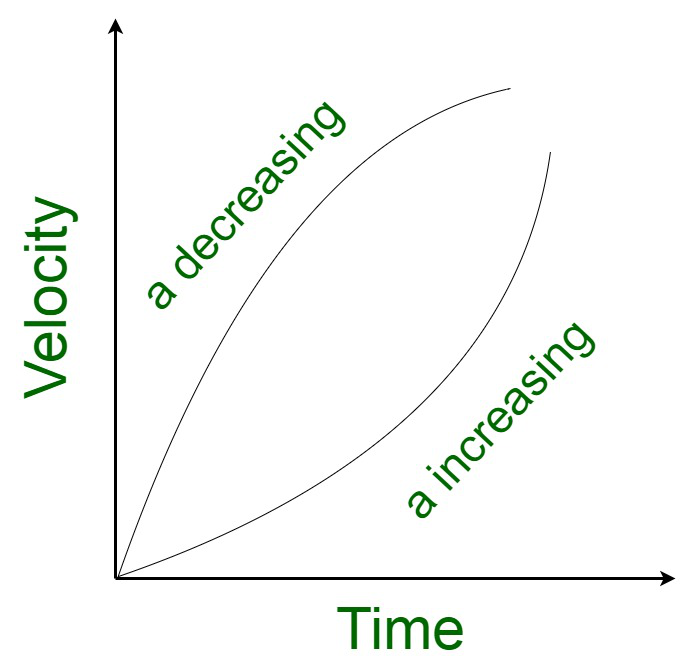• The total area enclosed by the time – velocity curve represents the distance travelled by a body.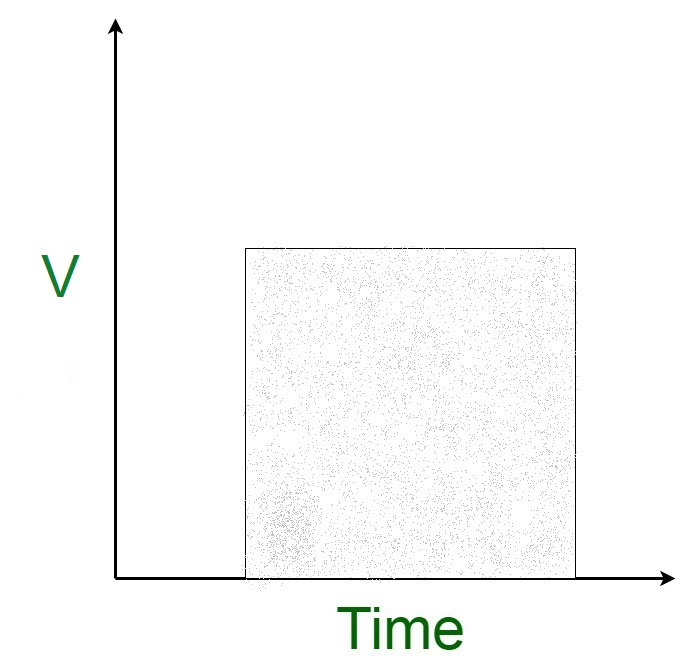Acceleration-Time graph

The curves indicate the acceleration-time graph where the time is plotted along the X-axis and acceleration is plotted along Y-axis. The following case may arise :

• When the acceleration of the particle is zero.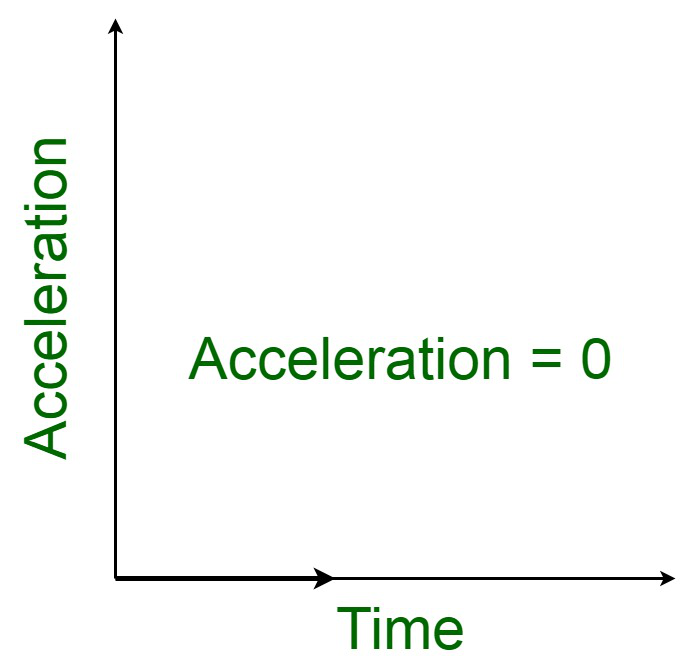• When acceleration is constant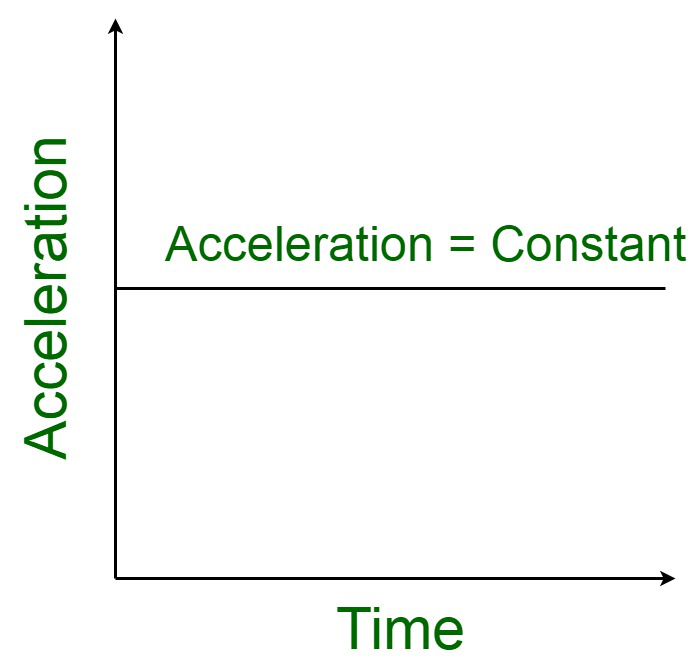• When acceleration is positively increasing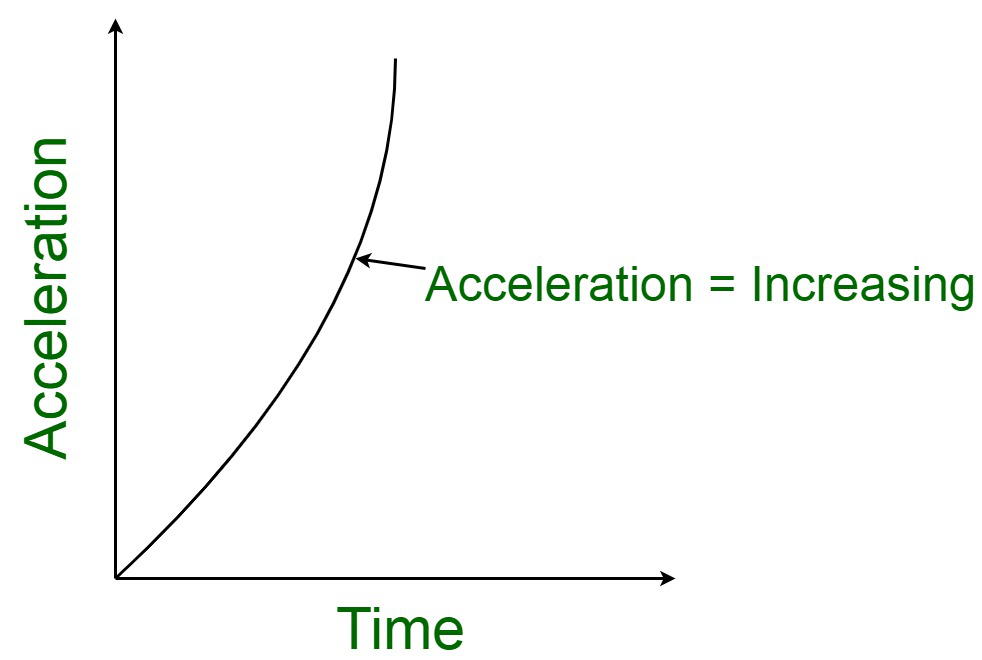• When acceleration is negatively decreasing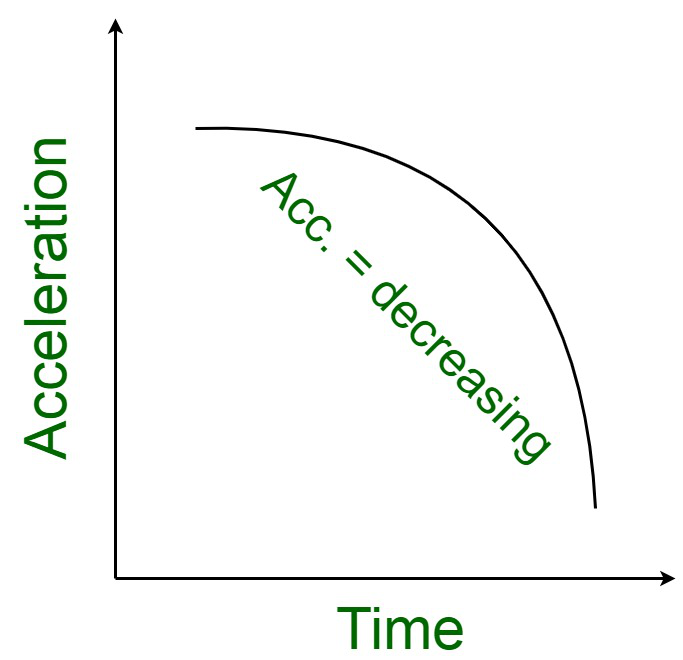• When initial acceleration is zero and rate of change of acceleration is non-uniform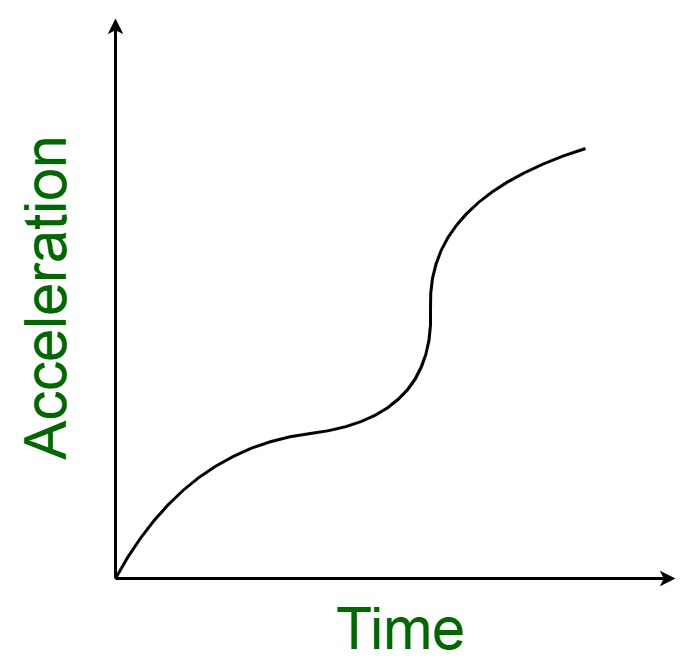• The change in velocity of the particle = area enclosed by the time-acceleration curve.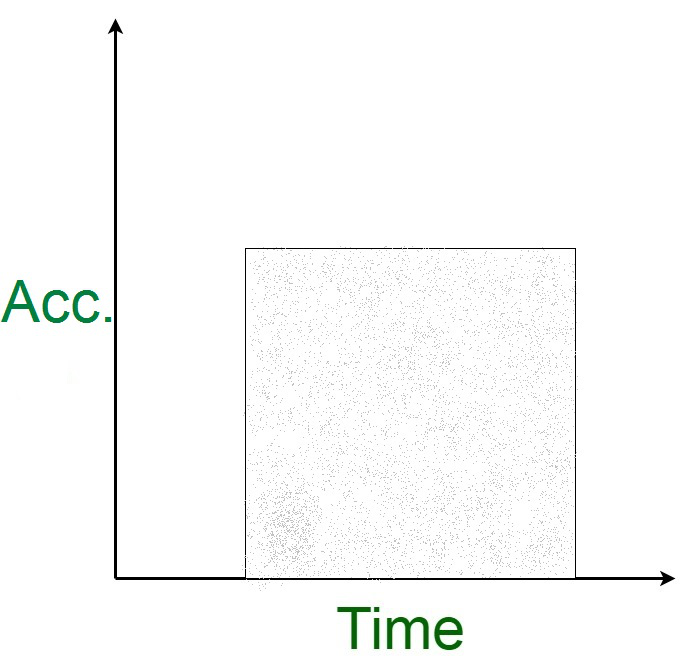Uniform acceleration

In case the velocity of an object changes in equal amounts during the same time interval, then the body is said to be in uniform acceleration.  In this case, neither the direction nor magnitude changes with respect to time.

Examples

• A ball rolling down the slope.
• When a bicycle rider is riding the bicycle on the slope  where both the pedals are engaged.
• A kid sliding down from the slider.
• Motion of car with constant velocity, etc..

Variable or non-uniform acceleration

Variable acceleration is the velocity of body that changes by varying amounts during same time interval. Variable acceleration comes into picture when the direction or magnitude or both of the object changes with respect to time.

Examples :

• A car changing speed
• Uniform circular motion
• Motion of pendulum with changing speed

Equations of Motion for Uniformly Accelerated Motion

For an object covering uniformly accelerated motion, the following equations derived can be used to relate displacement (x), time taken (f), initial velocity (u), final velocity (v) and acceleration (a).

The equation governs the final and initial velocities v and u of an object moving with uniform acceleration a: v = u + at, which can be visualised graphically as,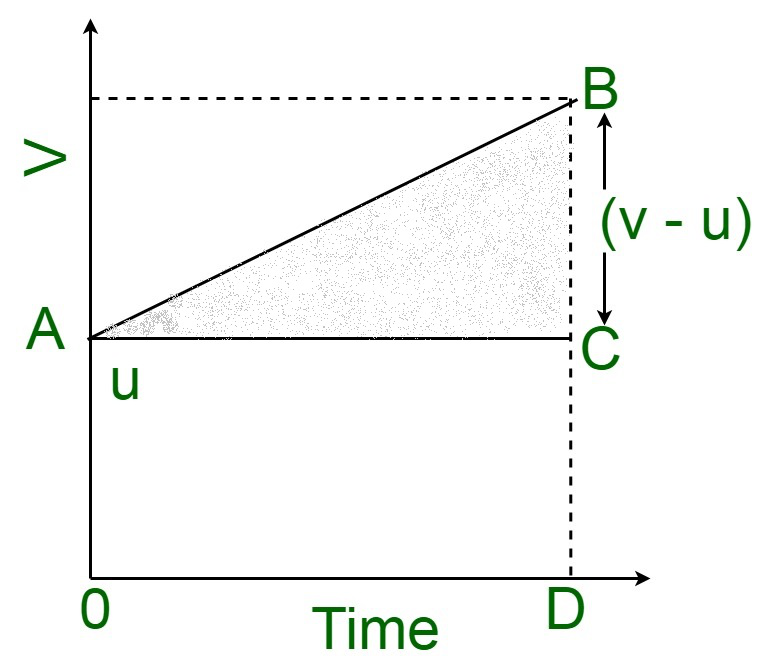The area under this curve is:

Area between the time instants, 0 and t are given by,

= Area of triangle ABC + Area of rectangle OACDNow,

The area under v – t curve represents the displacement.

Therefore, the displacement x of the object is:But, we have,

v – u = at

Therefore,or,The equation for displacement can also be given as follows:Earlier we have derived:

v = u + at

or,Substituting the value of t in the equation for displacement we get,So, we have derived following kinematic equation

v = u + at    ……..(i)Where,

u is initial velocity

v is final velocity

a is acceleration and

s is the distance covered in time interval t.

Displacement in a particular instant of time (nth second of the motion) is given by### Sample Problems

Problem 1: If a Truck accelerates from 6 m/s to 10 m/s in 10 s. Calculate its acceleration?

Solution:

Given that,

Initial Velocity u = 6 m/s,

Final Velocity v = 10 m/s,

Time taken t = 10 s.

We have to find Acceleration ‘a’

Acceleration, a = (v – u) / t

= (10 m/s – 6  m/s) / 10 s

= 0.4 m/s2

Thus, the acceleration of the truck is 0.4 m/s2.

Problem 2: If a ball is released from the terrace of a building to the ground. If the ball took 6 s to touch the ground. Find the height of the terrace from the ground?

Solution:

Given that,

Initial Velocity u = 0 {as the ball was at rest},

Time taken by the ball to touch the ground t = 6 seconds

Acceleration due to gravity a = g = 9.8 m/s2

Distance traveled by stone = Height of bridge  = s

The distance covered by the ball from the terrace to the groundTherefore,

Distance of the terrace from the ground is 29.4 m.

Problem 3: If a man is driving car at 108 km/h is slow down and bring to 72 km/h in 5 s. Calculate the retardation of the car?

Solution:

Given that,

Initial velocity u = 108 km/h

orFinal velocity v = 72 km/h

orTime taken = 5 seconds

Therefore, acceleration is,A negative sign shows retardation.

Problem 4: If a car moves from rest and then accelerates uniformly at the rate of 7.5 m/s2 for 10 s. Find the velocity of the train in 10 s.

Solution:

Given that,

Initial velocity u = 0 {as the car was at rest}

Acceleration a = 7.5 m/s2

Time t = 10 s

v = u + at

= 0 + 7.5 × 10

Problem 5: If an object moves along the x-axis according to the relation x = 1 – 2t + 3t2, where x is in meters and t is in seconds. Calculate the acceleration of the body when t = 3s.

Solution:

Given that,

x = 1 – 2t + 3t2

Velocity, v = dx/dt

= d/dt {1 – 2t + 3t2}

= -2 + 6t

Therefore, Acceleration a = dv/dt

= d/dt {-2 + 6t}

= 6 m/s

My Personal Notes arrow_drop_up